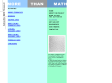Public marks with tag math:geometry http://blogmarks.net/marks/tag/math:geometry More Than Math http://www.morethanmath.org/activities.htm More Than Math integrates the visual arts into the 3rd, 4th and 5th grade mathematics curriculum using works of art to explore pattern, symmetry, proportion, perspective, balance and geometric form 2005-09-26T16:59:45Z cherbourg gr3, gr4, gr5, sesvt, ssdvt, math skills, math:geometry, math tool#### More Than Mathby cherbourg & 1 other (via)
More Than Math integrates the visual arts into the 3rd, 4th and 5th grade mathematics curriculum using works of art to explore pattern, symmetry, proportion, perspective, balance and geometric form
]]>